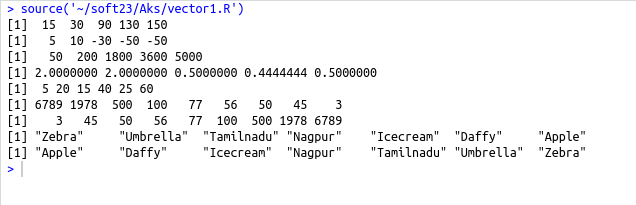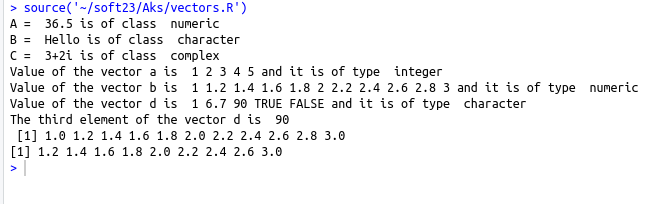• #5, First Floor, 4th Street Dr. Subbarayan Nagar Kodambakkam, Chennai-600 024 Landmark : Samiyar Madam
• pro@slogix.in
• +91- 81240 01111

### What is Vectors in R?

###### Description

To understand the vector concept and creation of single and multiple element vector.

###### Process

Vectors are the most basic R data objects and there are six types of atomic vectors. They are logical, integer, double, complex, character and raw.

Creating a single element vector :

Writing single value in R is taken as a vector of length 1 and belongs to any one class of the vector(i.e Numeric,character,complex,Logical) .

Creating a multiple element vector :

Multiples element vector can be created in different ways.

Using the colon (:) operator

Seq() function

C() function

Using the colon operator :

A=1:5

Using seq() :

b=seq(startvalue,endvalue,by=value)

By=value is the step size

Using c() :

a=c(5,7,TRUE,”ABC”,69.9)

Accessing vector :

Elements of a vector can be accessed by using [].

The index value starts from 1

Giving negative index drops the particular element

Vector arithmetic :

Two vectors can be added,subtracted,multiplied and divided whose output is also a vector

Vector element Recycling :

If we apply arithmetic operations to two vectors of unequal length, then the elements of the shorter vector are recycled to complete the operations.

The length of longer vector should be multiples of the length of shorter vector.

Vector sorting :

The elements of the vector can be sorted using the sort() function.

To sort the elements in descending order set the argument decreasing=TRUE

###### Sapmle Code

Source code : (Vector creation and accessing)

#single element vector
a=36.5
b=”Hello”
c=3+2i
cat(“A = “,a,”is of class “,class(a))
cat(“\nB = “,b,”is of class “,class(b))
cat(“\nC = “,c,”is of class “,class(c))
#muliple element vector
#colon operator
a=1:5
cat(“\nValue of the vector a is “,a,”and it is of type “,class(a))
#using seq()
b=seq(1,3,by=0.2)
cat(“\nValue of the vector b is “,b,”and it is of type “,class(b))
#using c()
d=c(1,6.7,90,”TRUE”,FALSE)
cat(“\nValue of the vector d is “,d,”and it is of type “,class(d))
#accessing vector using indexing
cat(“\nThe third element of the vector d is “,d,”\n”)
#using negative index drops the element
print(b)
print(b[c(-1,-10)])

Source code : (Vector arithmetic,element recycling,sorting)

#vector arithmetic
a=c(10,20,30,40,50)
b=c(5,10,60,90,100)
print(a+b)
print(a-b)
print(a*b)
print(a/b)
#vector element recycling
d=c(5,10,15,20,25,30)
e=c(1,2)
f=d*e
print(f)
#vector sorting
x=c(50,100,45,6789,3,56,77,1978,500)